﻿ 定位格架对燃料棒热工水力特性影响的研究 Study on the Influence of Positioning Lattice on Thermal Hydraulic Characteristics of Fuel Rod

Nuclear Science and Technology
Vol. 06  No. 04 ( 2018 ), Article ID: 27192 , 9 pages
10.12677/NST.2018.64012

Study on the Influence of Positioning Lattice on Thermal Hydraulic Characteristics of Fuel Rod

Cheng Lai, Jinchuan Bai, Cong Jin, Zhangnan Xu, Liang Yuan

Suzhou Thermal Research Institute, Shenzhen Guangdong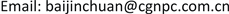Received: Sep. 28th, 2018; accepted: Oct. 13th, 2018; published: Oct. 20th, 2018ABSTRACT

Based on the ontology test of Benchmark, it was studied that how the positioning lattice spacing influenced on the critical heat flux and its occurrence point as well as the thermal hydraulic parameters of the critical passage. The results show that as the distance between the positioning lattice increases, the critical point moves upstream of the next positioning lattice, the critical heat flux increases, and the exit mass velocity of the critical passage decreases.

Keywords:Benchmark Test, Positioning Lattice, Critical Heat Flux1. 引言

2. 燃料组件结构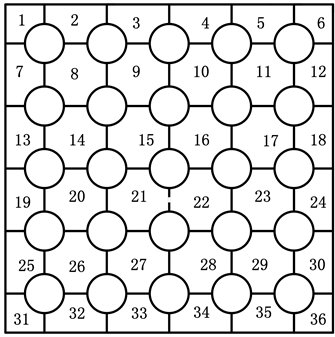Figure 1. 5 × 5 Component Subchannel Partition

3. 计算方法

3.1. 子通道划分

3.2. 计算模型

3.2.1. 子通道程序

1) 沸腾时，各子通道内的流体为一维两相可分离的滑移流模型。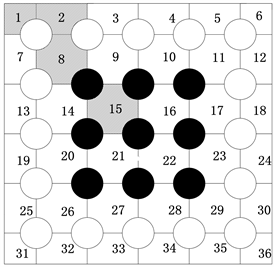Figure 2. Subchannel distribution diagram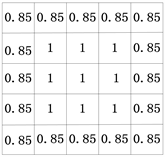Figure 3. Radial power distribution diagram

2) 空泡份额为焓，压力，质量流率，轴向位置和时间的函数。

3) 相邻子通道间的紊流交混不会引起流量的再分配。

4) 紊流交混可以叠加在能引起流量再分配的横流上。

5) 忽略声速传递的影响。

6) 在子通道内，横流速度远小于轴向速度。

${A}_{i}\frac{\partial {\rho }_{m,i}}{\partial t}+\frac{\partial {m}_{i}}{\partial x}=-\underset{j}{\sum }\left({w}_{ij}+{{w}^{\prime }}_{ij}\right)$

$\frac{{m}_{i}}{{u}^{″}}\frac{\partial {h}_{i}}{\partial t}+{m}_{i}\frac{\partial {h}_{i}}{\partial x}={q}^{\prime }-\underset{j}{\sum }{{w}^{\prime }}_{ij}\left({h}_{i}-{h}_{j}\right)-\underset{j}{\sum }c\left({t}_{i}-{t}_{j}\right)+{h}_{i}\underset{j}{\sum }{w}_{ij}-\underset{j}{\sum }{h}^{*}{w}_{ij}$

$\frac{1}{{A}_{i}}\frac{\partial {m}_{i}}{\partial t}-2{u}_{i}\frac{\partial {\rho }_{i}}{\partial t}+\frac{\partial \rho }{\partial x}={a}^{\prime }+\frac{2{u}_{i}\underset{j}{\sum }{w}_{ij}-\underset{j}{\sum }{u}^{*}{w}_{ij}}{{A}_{i}}$

${a}^{\prime }={\left(\frac{{m}_{i}}{{A}_{i}}\right)}^{2}\left(\frac{{v}_{i}f\varphi }{2D}+\frac{K{{v}^{\prime }}_{i}}{2\Delta x}+{A}_{i}\frac{\partial \left({{v}^{\prime }}_{i}/{A}_{i}\right)}{\partial x}+{\rho }_{i}g\mathrm{cos}\theta \right)-{f}_{T}\frac{\underset{j}{\sum }{{w}^{\prime }}_{ij}\left({u}_{i}-{u}_{j}\right)}{{A}_{i}}$

$\frac{\partial {w}_{ij}}{\partial t}+\frac{\partial {w}_{ij}{u}_{i}}{\partial x}=\frac{s}{l}c{w}_{ij}+\frac{s}{l}\left({P}_{i}-{P}_{j}\right)$

3.2.2. 计算流程图

3.3. 临界热流密度(CHF)关系式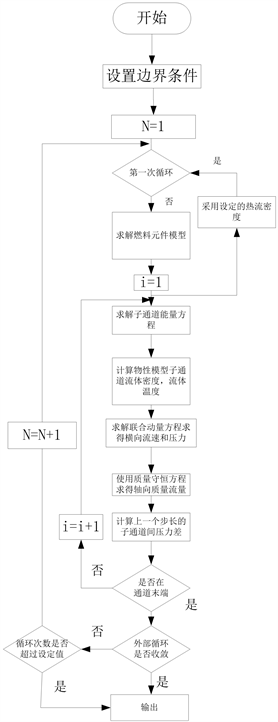Figure 4. The subchannel program calculates the flow chart

4. 计算结果与讨论

4.1. 临界热流密度计算方法比较

4.2. 定位格架间距对组件临界点的影响Figure 5. Comparison between the calculated value and the test value of the critical heat flux relation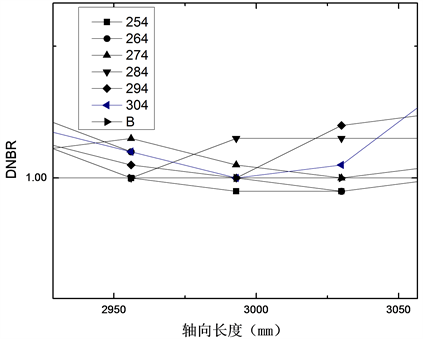Figure 6. MDNBR distribution under spacing change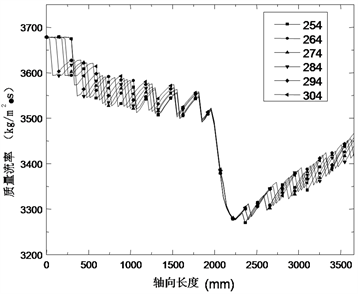Figure 7. Mass flow rate under spacing change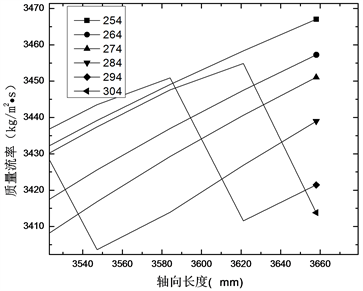Figure 8. Critical exit mass velocity under spacing change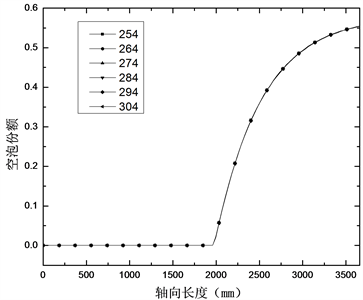Figure 9. Critical exit bubble under spacing change

5. 结论

1) 定位格架的存在加强了流体的交混。随着定位格架间距的增大，交混越充分，使得临界热流密度变大，同时临界点向下一个定位格架的上游移动。

2) 当定位格架的间距大于定位格架的交混长度时，组件临界热流密度和临界点的位置基本保持不变。

3) 随着定位格架间距的增大，发生临界的通道的质量流速沿轴线高度方向逐渐减小，且出口质量流速也相应变小。

Study on the Influence of Positioning Lattice on Thermal Hydraulic Characteristics of Fuel Rod[J]. 核科学与技术, 2018, 06(04): 99-107. https://doi.org/10.12677/NST.2018.64012

1. 1. 张龙根, 何玉枝, 朱炳艺. 压水堆燃料组件定位格架的交混长度[J]. 原子能科学技术, 1982, 16(3): 272.

2. 2. Rubin, A.J. (2011) OECD/NRC Benchmark Based on NUPEC PWR Subchannel and Bundle Test (PSBT)-Analysis Using Subchannel Code CTF and System Code Trace. Pennsylvania State University, State College.

3. 3. Jackson, J.W. and Cobra N.E. (1981) IIIc/MIT-2: A Digital Computer Program for Steady State and Transient Thermal-Hydraulic Analysis of Rod Bundle Nuclear Fuel Element. Todreas. Massachusetts Institute of Technology Cambridge, Cambridge.

4. 4. 张金玲, 郭玉君, 秋穗正, 等. 反应堆临界热流密度的计算[J]. 西安交通大学学报, 1995, 29(5): 118-123.

5. 5. 于平安, 朱瑞安, 喻真烷, 等. 核反应堆热工分析[M]. 上海: 上海交通大学出版社, 2002.